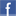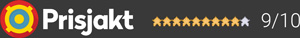#### Inga produkter tillagda!

Lägg en produkt i varukorgen så ser det lite roligare ut här ;)

#### Varukorg

0 kr# ArcamPA240

25 995 kr
Leverans 2-5 dagar ()
Fri frakt!

The PA240 is a high-performance power amplifier that delivers 2 channels of efficient Class G amplification. With an impressive 380W per channel, the PA240 is designed to offer the greatest flexibility and power without compromising on control.

Featuring the best-in-class components including a toroidal based power supply, acoustically damped chassis, paralleled transistor output stages and exceptionally low levels of distortion, the PA240 masters the reproduction of music with all its originality and detail for an unmatched level of sound quality and clarity.Dela på Facebook

## Specifikationer

Slutsteg
ModellPA240
TypHembioslutsteg
Effekt90 watt
Frekvensomfång20 - 20kHz +/-0.05dB Hz
THD (Total Harmonisk Distortion)80% power, 8Ω at 1kHz 0.001 %
AnslutningarRCA Type XLR Type
Strömförbrukning, standby/max<0.5/1.5k watt
Dimensioner (B x H x D)433x177x425 mm
Vikt18 kg

#### Frågor? Ring 031-241600eller maila //<![CDATA[ var l=new Array(); l = '>'; l = 'a'; l = '/'; l = '<'; l = '|109'; l = '|111'; l = '|99'; l = '|46'; l = '|110'; l = '|114'; l = '|97'; l = '|108'; l = '|108'; l = '|97'; l = '|107'; l = '|100'; l = '|117'; l = '|106'; l = '|108'; l = '|64'; l = '|111'; l = '|102'; l = '|110'; l = '|105'; l = '>'; l = '"'; l = '|109'; l = '|111'; l = '|99'; l = '|46'; l = '|110'; l = '|114'; l = '|97'; l = '|108'; l = '|108'; l = '|97'; l = '|107'; l = '|100'; l = '|117'; l = '|106'; l = '|108'; l = '|64'; l = '|111'; l = '|102'; l = '|110'; l = '|105'; l = ':'; l = 'o'; l = 't'; l = 'l'; l = 'i'; l = 'a'; l = 'm'; l = '"'; l = '='; l = 'f'; l = 'e'; l = 'r'; l = 'h'; l = ' '; l = 'a'; l = '<'; for (var i = l.length-1; i >= 0; i=i-1) { if (l[i].substring(0, 1) === '|') document.write("&#"+unescape(l[i].substring(1))+";"); else document.write(unescape(l[i])); } //]]>